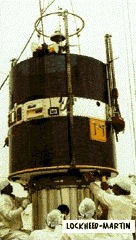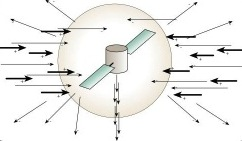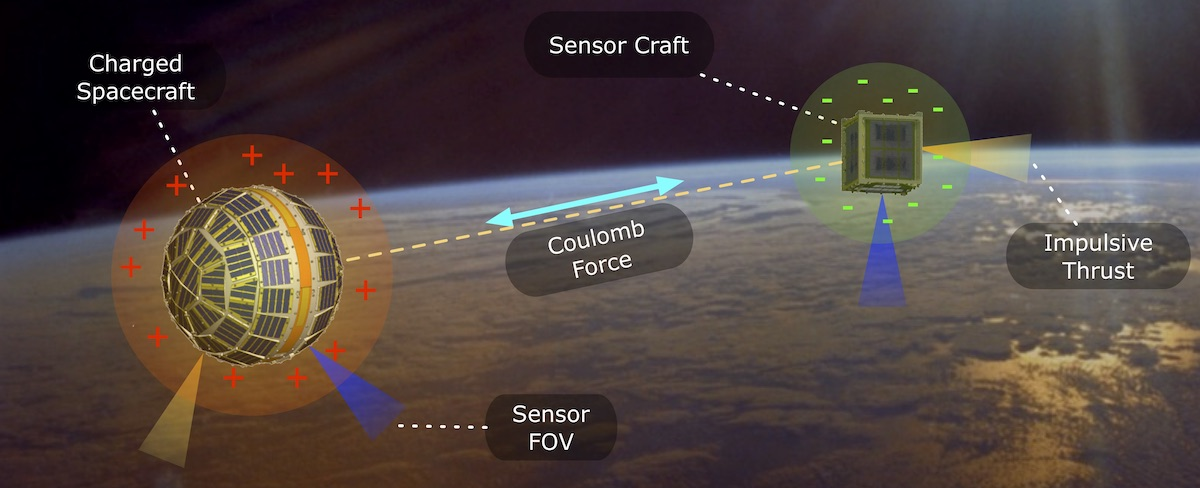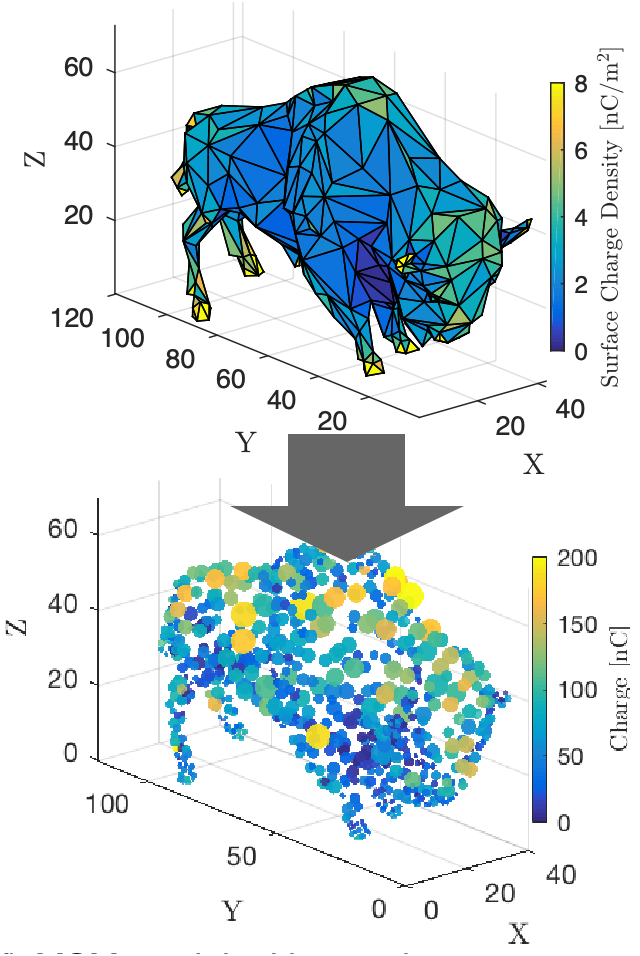# Coulomb Formation Flying

A GEO satellite isA GEO satellite is exposed to the space plasma environment which contains positively charged ions and negatively charged electrons. The faster electrons will accumulate more rapidly on the craft than the slower positively charged ions. This causes in a negative electric charge build-up to occur within the spacecraft. At steady-state charging conditions, the negative electric field about the spacecraft will repel a sufficiently large number of electrons such that a zero net current to the craft will result. Depending on the space plasma density, the steady-state charges can vary from near-zero to several kilovolts. In 1979 the SCATHA satellite was launched. One of its goals was to measure the build-up and breakdown of electrostatic charge on various spacecraft components, as well as to actively control the spacecraft charge using an electron beam. This mission was able to flight verify that it is possible to actively control the spacecraft charge. If another spacecraft had been present with a separation distance of about 20 meters, the natural uncontrolled SCATHA voltage levels would have been enough to impose inter-spacecraft forces in the milli-Newton level.The amount of electrical power required to generate these active electrical fields in less than 1 Watt. An ion engine operates by expelling charged particles (ions) at a very high velocity. The force generated is due to the momentum exchange between the particle and the spacecraft. The required large exit velocity of the ion particles results in the ion engines having a large electrical power requirement. To control the spacecraft charge, a comparable device to an ion engine would be used. However, here the ion exit velocity only would have to be large enough for the particle to escape the local electrical potential field. The massive reduction in required exit velocity results in a power requirement which is orders of magnitude smaller than that of an ion engine. The force exerted onto the Coulomb spacecraft due to momentum exchange with the expelled particle is negligible. The Coulomb satellite will only experience a force if additional charged spacecraft are in the vicinity. Similarly, the amount of mass expelled (charge ion particles) is so small that this mode of navigation control is referred to as being essentially propellant-less.;Note that such Coulomb forces will only control the relative motion of the satellite cluster, not the inertial motion of the cluster center of mass. For example, it would be impossible to use such Coulomb forces to boost the cluster orbit radius. However, it is possible to control the relative motion between the Coulomb satellites by changing the satellite charges. Thus, the Coulomb Formation Flying (CFF) concept allows for very fuel-efficient relative navigation with a very high control bandwidth. For example, a 1 meter spacecraft was found to be able to charge to 6 kV in as little as 8 ms using only 200 mW of power. The CFF concept could be used for general proximity flying (fly a sensor about a larger craft) or for controlling the relative motion of swarms or clusters of satellites. Since the magnitude of the Coulomb electrostatic force diminishes with 1/r2 of the separation distance, it is only effective for relatively tight formation/proximity flying scenario of 10-100 meters. For minimum separation distances larger than that, the required spacecraft charging levels simply become impractical. Further, the Coulomb force effectiveness is diminished in a high density space plasma environment. This is typically measured through the Debye length which indicates the exponential decay of the electrostatic field strength in a plasma environment. This decays is in addition to the natural point charge 1/r2 field strength reduction. For example, at LEO the Debye length is on the order of centimeters, thus preventing electrostatic forces from being an effective relative motion control method. At GEO, in comparison, the Debye length is between 100 and 1000 meters, depending on the current plasma density conditions.A particular challenge with Coulomb formation flying modeling is how to rapidly evaluate the electrostatic forces and torques. While a finite element solver can produce the answer within minutes, this is far too slow to perform dynamic simulation. Rather, solutions are required that can approximate the solution within a small fraction of a second. The Multi-Sphere-Method or MSM provides a computationally fast approximate model of a spacecraft’s electrostatic properties for applications that require faster-than-realtime results. MSM solves for the force and torque on a conductor by first finding the charge on a number of virtual spheres placed within the body. The charge on the spheres is found by assuming all spheres are equipotential across a single space object and solving a linear system of equations.MSM divides into the Volume MSM or VMSM, which uses a small number of spheres placed within the volume of the conductor, and the Surface MSM or SMSM, which places a large number of spheres on the surface of the conductor. It is more difficult to generate VMSM models because the size and location of the spheres must be found using an optimizer; however, VMSM models are much faster to run once completed due to the much smaller number of sphere employed. In contrast, SMSM models are easier to set up because the sphere locations are specified, and the sphere size can be found by matching self capacitance, which is computed from commercial FEM software. Generally, SMSM models show higher accuracy when compared to FEM-generated force and torque data.1 Although SMSM greatly reduces the effort required to create a MSM model for a given spacecraft geometry and increases the accuracy of the model, it comes at increased computational cost at runtime due to the large number of spheres. The latest research has been expanding the MSM modeling to account for time-varying shapes, di-electric material properties. The VMSM research has shown that even a 2-3 sphere model can provide very accurate E-field approximations at a few craft radii. For higher accuracy, a new heterogeneous SMSM model has been developed that allows the surface sphere locations to have different radii and separation distances to the other spheres. This new approach is developed from a Boundary Element based method and provides highly accurate approximations, but at a cost of computational speed in relation to the VMSM. The MSM models allow for rapid E-force and torque modeling to study the charged relative motion control such as in docking or close proximity operations. Further, these models are very useful to model the secondary electron emission about general shapes for the touchless sensing work.